# Linear-plateau response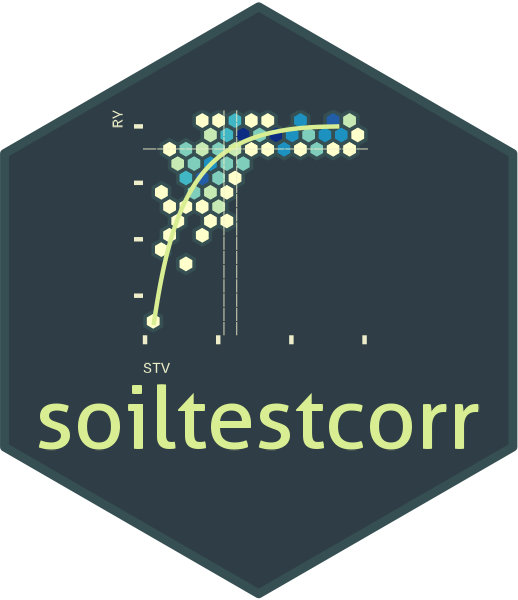## Description

This tutorial demonstrates the linear_plateau() function for fitting a continuous response model and estimating a critical soil test value (CSTV). This function fits a segmented regression model that follows two phases: a positive linear response and a flat plateau. The join point or break point is often interpreted as the CSTV. See Anderson and Nelson (1975) for examples.

$\begin{cases} x < j,\ y = a + bx \\ x > j,\ y = a + bj \end{cases}$

where
y represents the fitted crop relative yield,
x the soil test value,
a the intercept (ry when stv = 0),
b the slope (as the change in RY per unit of soil nutrient supply),
j the join point (a.k.a, break point) when the plateau phase starts (i.e., the CSTV).

The linear_plateau() function works automatically with self-starting initial values to facilitate the model’s convergence. The parameters of this regression model have simple interpretations.

• the crop relative yield response may be more curvilinear, such that a sharp break from linear response to plateau is unreasonable.

• the default CSTV confidence interval (based on symmetric Wald’s intervals) is generally unreliable. We recommend the user try the boot_linear_plateau() function for a reliable confidence interval estimation of parameters via bootstrapping (resampling with replacement).

## General Instructions

1. Load your data frame with soil test value and relative yield data.

2. Specify the following arguments in linear_plateau():

1. data (optional)

2. stv (soil test value)

3. ry (relative yield) columns or vectors

4. target (optional) for calculating the soil test value at some RY level along the slope segment.

5. tidy TRUE (produces a data.frame with results) or FALSE (store results as list)

6. plot TRUE (produces a ggplot as main output) or FALSE (no plot, only results as data.frame)

7. resid TRUE (produces plots with residuals analysis) or FALSE (no plot),

3. Run and check results.

4. Check residuals plot, and warnings related to potential limitations of this model.

5. Adjust curve plots as desired with additional ggplot2 functions.

# Tutorial

library(soiltestcorr)

Suggested packages

# Install if needed
library(ggplot2) # Plots
library(dplyr) # Data wrangling
library(tidyr) # Data wrangling
library(purrr) # Mapping

# Native fake dataset from soiltestcorr package
corr_df <- soiltestcorr::data_test

# Fit linear_plateau()

## 1. Individual fits

### 1.1. tidy = TRUE (default)

It returns a tidy data frame (more organized results)


linear_plateau(corr_df, STV, RY, tidy = TRUE)
#> # A tibble: 1 × 16
#>   inter…¹ slope equat…² plateau  CSTV lowerCL upperCL CI_type target  STVt   AIC
#>     <dbl> <dbl> <chr>     <dbl> <dbl>   <dbl>   <dbl> <chr>    <dbl> <dbl> <dbl>
#> 1    53.7  1.55 53.7 +…    96.2  27.4      24    30.7 Wald, …   96.2  27.4 1026.
#> # … with 5 more variables: AICc <dbl>, BIC <dbl>, R2 <dbl>, RMSE <dbl>,
#> #   pvalue <dbl>, and abbreviated variable names ¹​intercept, ²​equation

### 1.2. tidy = FALSE

It returns a LIST (may be more efficient for multiple fits at once)


linear_plateau(corr_df, STV, RY, tidy = FALSE)
#> $intercept #>  53.71661 #> #>$slope
#>  1.550917
#>
#> $equation #>  "53.7 + 1.55x when x < 27" #> #>$plateau
#>  96.18333
#>
#> $CSTV #>  27.38169 #> #>$lowerCL
#>  24
#>
#> $upperCL #>  30.7 #> #>$CI_type
#>  "Wald, 95%"
#>
#> $target #>  96.2 #> #>$STVt
#>  27.4
#>
#> $AIC #>  1025.96 #> #>$AICc
#>  1026.26
#>
#> $BIC #>  1037.64 #> #>$R2
#>  0.52
#>
#> $RMSE #>  9.94 #> #>$pvalue
#>  0

You can use the stv and ry vectors from the data frame using the $.  fit_vectors_tidy <- linear_plateau(stv = corr_df$STV, ry = corr_df$RY) fit_vectors_list <- linear_plateau(stv = corr_df$STV, ry = corr_df$RY, tidy = FALSE) ## 2. Multiple fits at once # Example 1. Fake dataset manually created data_1 <- data.frame("RY" = c(65,80,85,88,90,94,93,96,97,95,98,100,99,99,100), "STV" = c(1,2,3,4,5,6,7,8,9,10,11,12,13,14,15)) # Example 2. Native fake dataset from soiltestcorr package data_2 <- soiltestcorr::data_test # Example 3. Native dataset from soiltestcorr package, Freitas et al. (1966), used by Cate & Nelson (1971) data_3 <- soiltestcorr::freitas1966 %>% rename(STV = STK) data.all <- bind_rows(data_1, data_2, data_3, .id = "id") Note: the stv column needs to have the same name for all datasets if binding rows. ### 2.1. Using map()  # Run multiple examples at once with purrr::map() data.all %>% nest(data = c("STV", "RY")) %>% mutate(model = map(data, ~ linear_plateau(stv = .$STV, ry = .$RY))) %>% unnest(model) #> # A tibble: 3 × 18 #> id data intercept slope equation plateau CSTV lowerCL upperCL CI_type #> <chr> <list> <dbl> <dbl> <chr> <dbl> <dbl> <dbl> <dbl> <chr> #> 1 1 <tibble> 65.9 5.09 65.9 + 5… 97.4 6.21 5.1 7.4 Wald, … #> 2 2 <tibble> 53.7 1.55 53.7 + 1… 96.2 27.4 24 30.7 Wald, … #> 3 3 <tibble> 39.2 0.752 39.2 + 0… 95.6 75.0 46.4 104. Wald, … #> # … with 8 more variables: target <dbl>, STVt <dbl>, AIC <dbl>, AICc <dbl>, #> # BIC <dbl>, R2 <dbl>, RMSE <dbl>, pvalue <dbl> ### 2.2. Using group_modify() Alternatively, with group_modify, nested data is not required. However, it still requires a grouping variable (in this case, id) to identify each dataset. group_map() may also be used, though list_rbind() is required to return a tidy data frame of the model results instead of a list.  data.all %>% group_by(id) %>% group_modify(~ linear_plateau(data = ., STV, RY)) #> # A tibble: 3 × 17 #> # Groups: id  #> id inter…¹ slope equat…² plateau CSTV lowerCL upperCL CI_type target STVt #> <chr> <dbl> <dbl> <chr> <dbl> <dbl> <dbl> <dbl> <chr> <dbl> <dbl> #> 1 1 65.9 5.09 65.9 +… 97.4 6.21 5.1 7.4 Wald, … 97.4 6.2 #> 2 2 53.7 1.55 53.7 +… 96.2 27.4 24 30.7 Wald, … 96.2 27.4 #> 3 3 39.2 0.752 39.2 +… 95.6 75.0 46.4 104. Wald, … 95.6 75 #> # … with 6 more variables: AIC <dbl>, AICc <dbl>, BIC <dbl>, R2 <dbl>, #> # RMSE <dbl>, pvalue <dbl>, and abbreviated variable names ¹​intercept, #> # ²​equation ## 3. Bootstrapping Bootstrapping is a suitable method for obtaining confidence intervals for parameters or derived quantities. Bootstrapping is a resampling technique (with replacement) that draws samples from the original data with the same size. If you have groups within your data, you can specify grouping variables as arguments in order to maintain, within each resample, the same proportion of observations than in the original dataset. This function returns a table with as many rows as the resampling size (n) containing the results for each resample. set.seed(123) boot_lp <- boot_linear_plateau(corr_df, STV, RY, n = 500) # only 500 for sake of speed boot_lp %>% head(n = 5) #> # A tibble: 5 × 13 #> boot_id inter…¹ slope plateau CSTV target STVt AIC AICc BIC R2 RMSE #> <dbl> <dbl> <dbl> <dbl> <dbl> <dbl> <dbl> <dbl> <dbl> <dbl> <dbl> <dbl> #> 1 1 49.0 1.72 96.2 27.4 96.2 27.4 1028. 1029. 1040. 0.55 10.0 #> 2 2 56.4 1.38 95.9 28.5 95.9 28.5 1015. 1015. 1027. 0.49 9.55 #> 3 3 62.6 1.12 96.2 29.9 96.2 29.9 1004. 1004. 1016. 0.43 9.17 #> 4 4 60.0 1.22 97.1 30.4 97.1 30.4 992. 992. 1003. 0.56 8.77 #> 5 5 61.2 1.24 96.5 28.6 96.5 28.6 978. 979. 990. 0.51 8.35 #> # … with 1 more variable: pvalue <dbl>, and abbreviated variable name #> # ¹​intercept # CSTV Confidence Interval quantile(boot_lp$CSTV, probs = c(0.025, 0.5, 0.975))
#>     2.5%      50%    97.5%
#> 22.61863 27.60057 32.16080

# Plot
boot_lp %>%
ggplot(aes(x = CSTV))+
geom_histogram(color = "grey25", fill = "#9de0bf", bins = 10)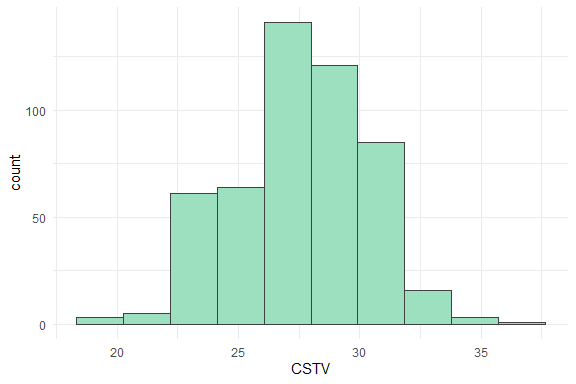## 4. Plots

### 4.1. Correlation Curve

We can generate a ggplot with the same linear_plateau() function. We just need to specify the argument plot = TRUE.

data_3 <- soiltestcorr::freitas1966

plot_lp <- linear_plateau(data = data_3, STK, RY, plot = TRUE)

plot_lp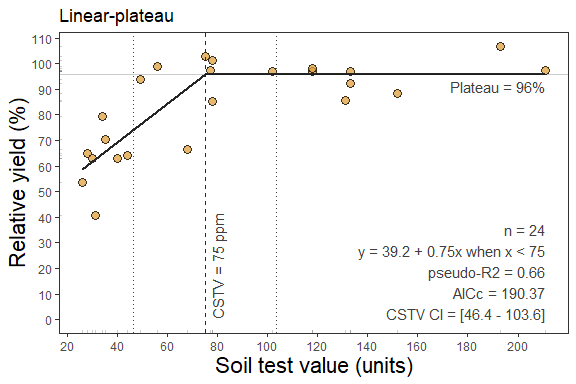### 4.2. Fine-tune the plots

As ggplot object, plots can be adjusted in several ways, such as modifying titles and axis scales.

plot_lp +
# Main title
ggtitle("My own plot title")+
# Axis titles
labs(x = "Soil Test K (ppm)",
y = "Cotton RY(%)") +
# Axis scales
scale_x_continuous(limits = c(20,220),
breaks = seq(0,220, by = 10)) +
# Axis limits
scale_y_continuous(limits = c(30,100),
breaks = seq(30,100, by = 10))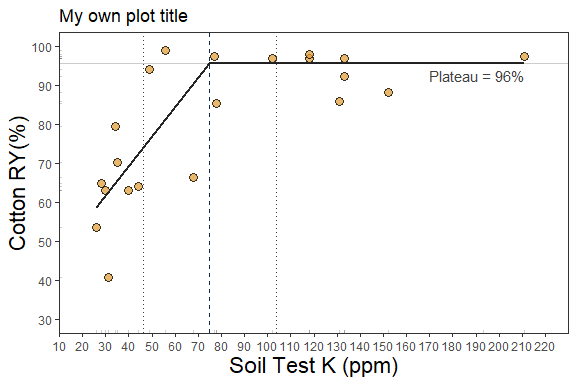### 4.3. Residuals

Set argument resid = TRUE.


# Residuals plot

linear_plateau(data_3, STK, RY, resid = TRUE)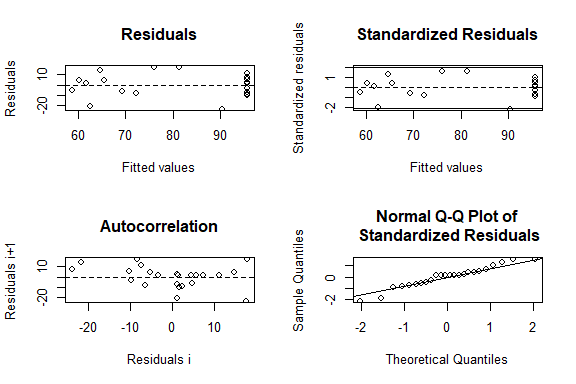#> # A tibble: 1 × 16
#>   inter…¹ slope equat…² plateau  CSTV lowerCL upperCL CI_type target  STVt   AIC
#>     <dbl> <dbl> <chr>     <dbl> <dbl>   <dbl>   <dbl> <chr>    <dbl> <dbl> <dbl>
#> 1    39.2 0.752 39.2 +…    95.6  75.0    46.4    104. Wald, …   95.6    75  188.
#> # … with 5 more variables: AICc <dbl>, BIC <dbl>, R2 <dbl>, RMSE <dbl>,
#> #   pvalue <dbl>, and abbreviated variable names ¹​intercept, ²​equation